This is an excerpt from the Python Data Science Handbook by Jake VanderPlas; Jupyter notebooks are available on GitHub.

The text is released under the CC-BY-NC-ND license, and code is released under the MIT license. If you find this content useful, please consider supporting the work by buying the book!

# Simple Scatter Plots

Another commonly used plot type is the simple scatter plot, a close cousin of the line plot. Instead of points being joined by line segments, here the points are represented individually with a dot, circle, or other shape. We’ll start by setting up the notebook for plotting and importing the functions we will use:

In :
%matplotlib inline
import matplotlib.pyplot as plt
plt.style.use('seaborn-whitegrid')
import numpy as np


## Scatter Plots with plt.plot¶

In the previous section we looked at plt.plot/ax.plot to produce line plots. It turns out that this same function can produce scatter plots as well:

In :
x = np.linspace(0, 10, 30)
y = np.sin(x)

plt.plot(x, y, 'o', color='black');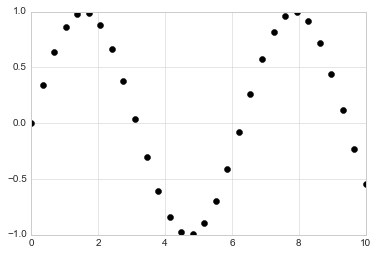The third argument in the function call is a character that represents the type of symbol used for the plotting. Just as you can specify options such as '-', '--' to control the line style, the marker style has its own set of short string codes. The full list of available symbols can be seen in the documentation of plt.plot, or in Matplotlib's online documentation. Most of the possibilities are fairly intuitive, and we'll show a number of the more common ones here:

In :
rng = np.random.RandomState(0)
for marker in ['o', '.', ',', 'x', '+', 'v', '^', '<', '>', 's', 'd']:
plt.plot(rng.rand(5), rng.rand(5), marker,
label="marker='{0}'".format(marker))
plt.legend(numpoints=1)
plt.xlim(0, 1.8);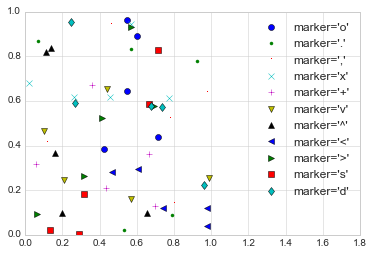For even more possibilities, these character codes can be used together with line and color codes to plot points along with a line connecting them:

In :
plt.plot(x, y, '-ok');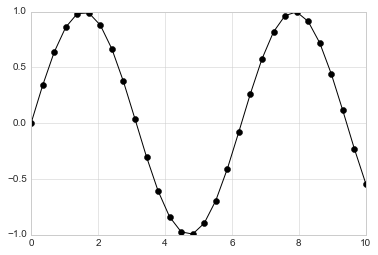Additional keyword arguments to plt.plot specify a wide range of properties of the lines and markers:

In :
plt.plot(x, y, '-p', color='gray',
markersize=15, linewidth=4,
markerfacecolor='white',
markeredgecolor='gray',
markeredgewidth=2)
plt.ylim(-1.2, 1.2);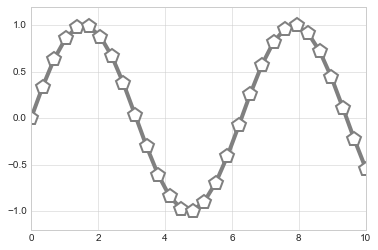This type of flexibility in the plt.plot function allows for a wide variety of possible visualization options. For a full description of the options available, refer to the plt.plot documentation.

## Scatter Plots with plt.scatter¶

A second, more powerful method of creating scatter plots is the plt.scatter function, which can be used very similarly to the plt.plot function:

In :
plt.scatter(x, y, marker='o');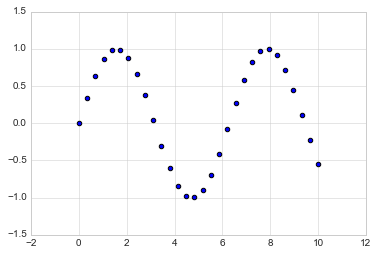The primary difference of plt.scatter from plt.plot is that it can be used to create scatter plots where the properties of each individual point (size, face color, edge color, etc.) can be individually controlled or mapped to data.

Let's show this by creating a random scatter plot with points of many colors and sizes. In order to better see the overlapping results, we'll also use the alpha keyword to adjust the transparency level:

In :
rng = np.random.RandomState(0)
x = rng.randn(100)
y = rng.randn(100)
colors = rng.rand(100)
sizes = 1000 * rng.rand(100)

plt.scatter(x, y, c=colors, s=sizes, alpha=0.3,
cmap='viridis')
plt.colorbar();  # show color scale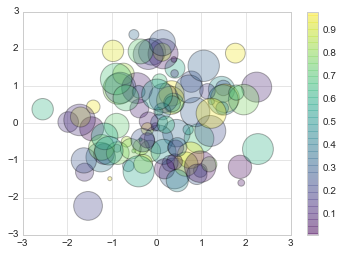Notice that the color argument is automatically mapped to a color scale (shown here by the colorbar() command), and that the size argument is given in pixels. In this way, the color and size of points can be used to convey information in the visualization, in order to visualize multidimensional data.

For example, we might use the Iris data from Scikit-Learn, where each sample is one of three types of flowers that has had the size of its petals and sepals carefully measured:

In :
from sklearn.datasets import load_iris
features = iris.data.T

plt.scatter(features, features, alpha=0.2,
s=100*features, c=iris.target, cmap='viridis')
plt.xlabel(iris.feature_names)
plt.ylabel(iris.feature_names);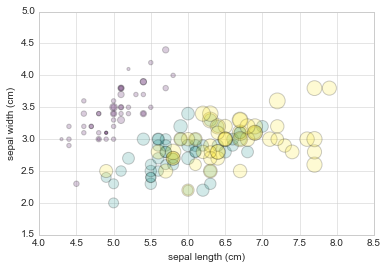We can see that this scatter plot has given us the ability to simultaneously explore four different dimensions of the data: the (x, y) location of each point corresponds to the sepal length and width, the size of the point is related to the petal width, and the color is related to the particular species of flower. Multicolor and multifeature scatter plots like this can be useful for both exploration and presentation of data.

## plot Versus scatter: A Note on Efficiency¶

Aside from the different features available in plt.plot and plt.scatter, why might you choose to use one over the other? While it doesn't matter as much for small amounts of data, as datasets get larger than a few thousand points, plt.plot can be noticeably more efficient than plt.scatter. The reason is that plt.scatter has the capability to render a different size and/or color for each point, so the renderer must do the extra work of constructing each point individually. In plt.plot, on the other hand, the points are always essentially clones of each other, so the work of determining the appearance of the points is done only once for the entire set of data. For large datasets, the difference between these two can lead to vastly different performance, and for this reason, plt.plot should be preferred over plt.scatter for large datasets.